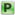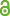Hauptmenü
• Autor
• Elsholtz, Christian
• Planitzer, Stefan
• TitelOn Erdős and Sárközy's sequences with Property P
• Datei
• DOI10.1007/s00605-016-0995-9
• Persistent Identifier
• Erschienen inMonatshefte für Mathematik
• Band182
• Erscheinungsjahr2017
• Heft3
• Seiten565-575
• ISSN1436-5081
• Zugriffsrechte• AbstractA sequence A of positive integers having the property that no element $$a_i \in A$$ divides the sum $$a_j+a_k$$ of two larger elements is said to have `Property P'. We construct an infinite set $$S \subset \mathbb{N}$$ having Property P with counting function $$S(x) \gg \frac{\sqrt{x}}{\sqrt{\log x}(\log\log x)^2(\log\log\log x)^2}$$ . This improves on an example given by Erdős and Sárközy with a lower bound on the counting function of order $$\frac{\sqrt{x}}{\log x}$$.